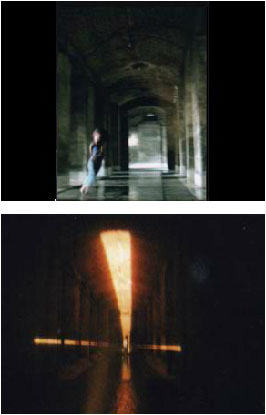ULISES CONTI WORKS BIO CONTACT34 Metros Dance Directed by: Diana Szeinblum and Luciano Suardi CETC (Centro de Experimentación del Teatro Colón) Soundtrack 34 meters = 0.0065 leagues = 28.56 yards = how many light-years? = 0.0229 miles = 111 feet = how many hands of mine in your back? = 1338 inches = 34 meters = 334 hands = how far is it when you look at me? = 75 cubits = 151 hand spans = 17 orgies = how many of your absences? = 34 meters = 0.18 stadiums = 0.031 walk in a resting day = how many minutes? What is the size of your things?